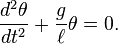# Period and Tension of a Pendulum

• postfan
T)## is a solution to this equation. If it is, then the period is T. Otherwise, the period is twice the original value.In summary, The tension in a pendulum is maximum when the pendulum is at the angle θg where θ_g=θ_0.f

## Homework Statement

A simple pendulum experiment is constructed from a point mass m attached to a pivot by a massless rod of length
L in a constant gravitational field. The rod is released from an angle θ0 < π/2 at rest and the period of motion is
found to be T0. Ignore air resistance and friction.
19. At what angle θg during the swing is the tension in the rod the greatest?
(A) The tension is the greatest at the point θ_g = θ_0.
(B) The tension is the greatest at the point θ_g = 0.
(C) The tension is the greatest at an angle θ_g with 0 < θ_g < θ_0.
(D) The tension is constant.
(E) None of the above is true for all values of θ0 with 0 < θ0 < π/2.
20. What is the maximum value of the tension in the rod?
(A) mg
(B) 2mg
(C) mLθ0_/T_0^2
(D) mg sin θ_0
(E) mg(3 − 2 cos θ_0)
21. The experiment is repeated with a new pendulum with a rod of length 4L, using the same angle θ0, and the period of motion is found to be T. Which of the following statements is correct?
(A) T = 2T_0 regardless of the value of θ_0.
(B) T > 2T_0 with T ≈ 2T_0 if θ_0 << 1.
(C) T < 2T_0 with T ≈ 2T_0 if θ_0 << 1.
(D) T > 2T_0 for some values of θ_0 and T < 2T_0 for other values of θ_0.
(E) T_0 and T are undefined because the motion is not periodic unless θ_0 << 1.

## The Attempt at a Solution

For 19, I just drew an FBD and realized that the tension in a pendulum is just mgcos(θ) which is maximized when θ=0 so the answer is B.
For 20, when θ=0 (where the maximum tension is) from the formula the tension is just mg which is (A).
For 21, I used the formula T=2pi*sqrt(L/g) so quadrupling the length should double the period making it (A).

Are my answers (and most importantly my justifications) right?

For 19, I just drew an FBD and realized that the tension in a pendulum is just mgcos(θ) which is maximized when θ=0 so the answer is B.
For 20, when θ=0 (where the maximum tension is) from the formula the tension is just mg which is (A).
For 21, I used the formula T=2pi*sqrt(L/g) so quadrupling the length should double the period making it (A).

Are my answers (and most importantly my justifications) right?

19 and 21 are correct.

For 20, you'll need to find the velocity of the bob at the lowest point. The tension provides the necessary centripetal force for the semi-circular motion, so consider that as well.

FC = mv2/r

For 19, I just drew an FBD and realized that the tension in a pendulum is just mgcos(θ)
You're overlooking centripetal acceleration. The rod not only has to counter gravity in the direction of the rod shaft, it also needs to provide the centripetal acceleration needed to make the bob travel in an arc. It doesn't change the answer to 19, but
when θ=0 (where the maximum tension is) from the formula the tension is just mg
it does change the answer to 20.
For 21, I used the formula T=2pi*sqrt(L/g)
That formula is only valid for small oscillations. Here θ0 can be up to π/2, which is definitely not small.

Ok so basically if T-mg=mv^/r then T=mg+mv^/r , but how do I find v^2/r in terms of g and a trig function?

Also how do you find the period with large oscillations?

Ok so basically if T-mg=mv^/r then T=mg+mv^/r , but how do I find v^2/r in terms of g and a trig function?

At the initial position, the pendulum will possesses potential energy. This will all be converted to kinetic evergy at the lowermost position. Get the value of 'v' using this.

Last edited:
Also how do you find the period with large oscillations?
In general, that's a hard problem. Fortunately, all you care about here is how varying the length affects the period, keeping the angle fixed.
Can you write down the general differential equation for a simple pendulum (not using any small angle approximation)?

Ok for 20 I did mg(L-Lcos(theta))=.5mv^2 and got v^2=2gL(1-cos(theta)). Substituting that into my "T" equation I got T=mg(3-2cos(theta)). How is that ?
For 21Ok for 20 I did mg(L-Lcos(theta))=.5mv^2 and got v^2=2gL(1-cos(theta)). Substituting that into my "T" equation I got T=mg(3-2cos(theta)). How is that ?

Yes! You got that right, mate..!